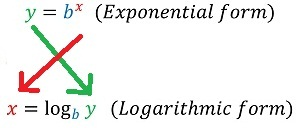Don't have an account? Register now to chat, post, use our tools, and much more.
I need help on reversing a formula for one of my projects. If you go to the website, I kind of already explained what I need.

https://imgur.com/a/JdtMt
Here's the image:You should look that up on Khan Academy.
I'd really appreciate it if someone actually solved the equation. I don't recall learning how to do that on khan academy (that's where I learned algebra before I was in the GRADE to learn algebra). I know how to solve equations with roots and powers, just not with two variables. I moved the 25 over to the other side (2^(X/2)=Y/25) and got stuck. I'm not sure how to describe Y in terms of x. I know that what I wanted was to find the relationship between {2,4,6,8,10} and {50,100,200,400,800}. Just did the exact opposite of what I needed.

Edit: Here's my work: X=50| (x/2 = 2^1) X=100| (x/2 = 2^2) X=200| (x/2 = 2^3)
I recognize the pattern, but I can't correlate X|{50,100,200,400,800} with Y|{1,2,3,4,5} from x to y instead of y to x; The same problem I initially started with!
All right so you have
25*2^(x/2)=y
and you want to solve for x.
You already moved the 25 over so now it's
2^(x/2)=(y/25)
what's 2^(x/2) equal to?
²√(2^x) this is a square root (since the bottom of the fractional exponent was a 2 [if it was a 3, then it would create a cube root]) to the power of x (since the numerator of the fractional exponent was a x)
so now you have
√(2^x) = (y/25)
Now this is where I'm not entirely sure which is correct to do next. I belive you need to square both sides to make
2^x=(y/25)^2
then you need to take the log of 2^x which makes
x=Log(Base:2)((y/25)^2)

I think this is correct, it seems to graph correctly!

Please reply if something doesn't make sense.LogBase? Hmm.. I assumed the answer would simply be in a root. After all, I only had a power in my original formula

Edit: Ran the logbase through my calculator... something's not right here...
TI84plceBasicPro wrote:
LogBase? Hmm.. I assumed the answer would simply be in a root. After all, I only had a power in my original formula

Edit: Ran the logbase through my calculator... something's not right here...

The LogBase command is found in the Math menu, and takes two arguments.

Here's the wiki page: http://tibasicdev.wikidot.com/logbase
To solve an exponential formula (where the X is in the exponent), you need logs. In algebra courses, they don't go into how to actually solve a logarithm, you simply need to transform to its logarithmic form.
Here is how to convert from one form to the otherOnce you have it in this form, for algebra courses, you are expected to use a calculator from there.
To actually solve equations in logarithmic form manually (assuming it isn't one of the basic ones that you would know in your head), you need calculus.
Here are the main series if you want to delve into it:On the ti-84 calcs, the logBASE command allows you to solve a log. Obviously it can't do any fancy calculus and has to resort to numerical methods. I believe it uses a combination of the CORDIC algorithm with a lookup table, which converges at one bit per iteration, so it takes a fixed constant number of iterations to compute any log.
Try this formula

x = ln(y/25) / ln(2) * 2

ln (lower case L n) should be found in the catalog (log of n).
I already figured that out. Thanks for all you're help guys though. :D

TheLastMillenial was right about x=logBASE 2 (y/25). I forgot that it was (x/2)=logBASE 2 (y/25)^2 instead of x=logBASE 2 (y/25).

Edit: I'm not really sure if that was worth all that trouble, especially since I took all night to code it so when you entered a number it would round it down to the nearest you could afford:

Code:
``` ... Input "PAY (MONEY): ",J If J=0:M→J If J>800:800→J J→Ś logBASE((J/25)²,2)→L iPart(L)→L If fPart(L/2)≠0:L-1→L 25(2^(L/2))→J Output(1,14,J ... ```

Register to Join the Conversation
Have your own thoughts to add to this or any other topic? Want to ask a question, offer a suggestion, share your own programs and projects, upload a file to the file archives, get help with calculator and computer programming, or simply chat with like-minded coders and tech and calculator enthusiasts via the site-wide AJAX SAX widget? Registration for a free Cemetech account only takes a minute.

»
» All times are GMT - 5 Hours

You cannot post new topics in this forum
You cannot reply to topics in this forum
You cannot edit your posts in this forum
You cannot delete your posts in this forum
You cannot vote in polls in this forum